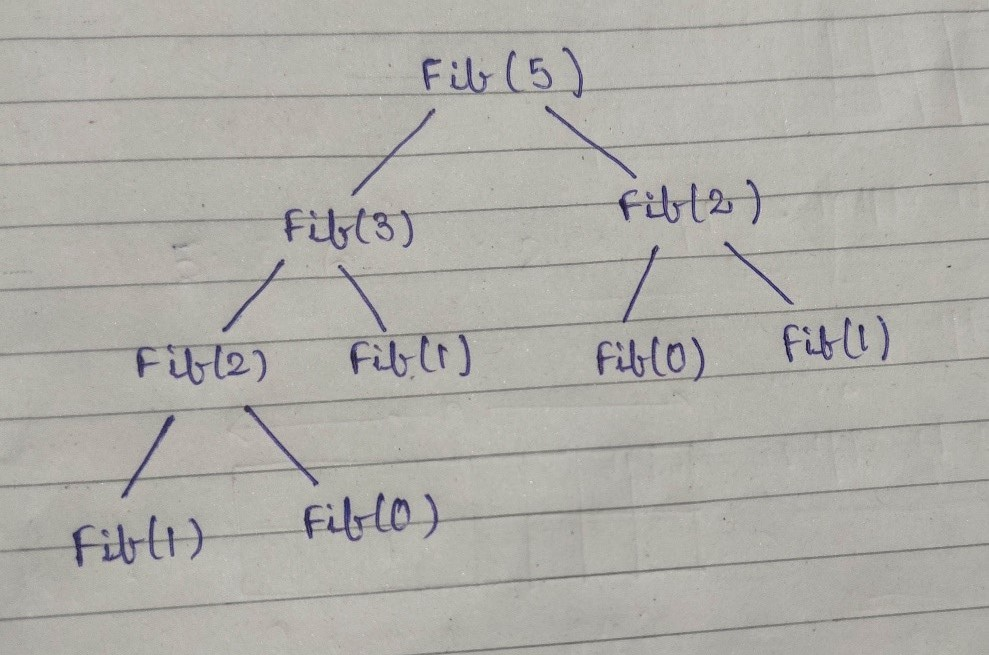Top Down and Bottom Up Approach

There are two approaches in dynamic programming:

1. Top-Down Approach

2. Bottom-Up Approach

Top Down Approach

· As the name suggests we are starting from top and moving downwards.

· We assume that the result of the subproblems are already calculated and if not calculated then we calculate them first.

· Let’s take a very common example of calculating Fibonacci numbers and understand this approach :. Suppose we are calculating 5th Fibonacci number. We know the recursive condition is fib(n)=fib(n-1)+fib(n-2), where fib(0)=fib(1)=1.

· Now in Top Down approach we will start from the top assuming that we can get the answer from the top. But since we do not know fib(5) in the starting, we will go downwards and look for fib(4) and fib(3).

· This process will continue until we reach the base condition , that are, fib(0)=fib(1)=1.

· Then we will backtrack and fill their parent nodes and finally we will get the value of fib(5).

Code :

Bottom Up Approach

· As the name suggests that we are starting from base and then moving forward/upwards.

· It is just the reverse process of Top Down Approach. We will start with the base values and then fill the dp table/array upwards.

· For example, we first calculate fib(0) then fib(1) then fib(2) then fib(3) and so on.

· Sometimes we use a term tabulation for this process because it’s like we fill a table from start.

Code :

Practice Problems :

Happy Coding 😊

By Programmers Army

Contributed by Shubham Kumar